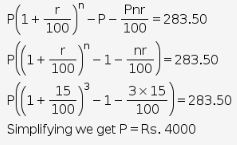# The difference between the compound interest and the simple interest

The difference between the compound interest and the simple interest on a certain sum at the rate of 15% per annum for 3 years is ₹283.50. Find the sum.

According to teh question,
n = 3 years, r = 15% p.a. and
difference between compound and simple interest = Rs. 283.50